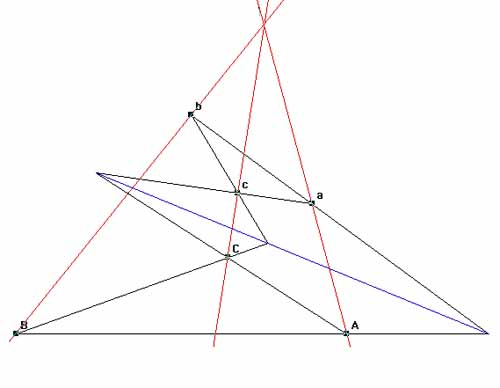# Desargues' theorem on perspective trianglesTriangles $ABC$ and $abc$ are said to be in perspective if the joins of corresponding points are concurrent.
[i.e. The lines $Aa, Bb, Cc$ meet at a point.]

For such triangles the meets of corresponding sides are collinear.
[i.e. $AB \bigcap ab, AC \bigcap ac, BC \bigcap bc$ lie on a straight line.]

This can be summarised as:

Triangles which are in perspective from a point are in perspective from a line.

Last Updated August 2020